Test: Set Theory & Algebra- 2

# Test: Set Theory & Algebra- 2 - Electrical Engineering (EE)

Test Description

## 15 Questions MCQ Test GATE Electrical Engineering (EE) 2024 Mock Test Series - Test: Set Theory & Algebra- 2

Test: Set Theory & Algebra- 2 for Electrical Engineering (EE) 2023 is part of GATE Electrical Engineering (EE) 2024 Mock Test Series preparation. The Test: Set Theory & Algebra- 2 questions and answers have been prepared according to the Electrical Engineering (EE) exam syllabus.The Test: Set Theory & Algebra- 2 MCQs are made for Electrical Engineering (EE) 2023 Exam. Find important definitions, questions, notes, meanings, examples, exercises, MCQs and online tests for Test: Set Theory & Algebra- 2 below.
Solutions of Test: Set Theory & Algebra- 2 questions in English are available as part of our GATE Electrical Engineering (EE) 2024 Mock Test Series for Electrical Engineering (EE) & Test: Set Theory & Algebra- 2 solutions in Hindi for GATE Electrical Engineering (EE) 2024 Mock Test Series course. Download more important topics, notes, lectures and mock test series for Electrical Engineering (EE) Exam by signing up for free. Attempt Test: Set Theory & Algebra- 2 | 15 questions in 45 minutes | Mock test for Electrical Engineering (EE) preparation | Free important questions MCQ to study GATE Electrical Engineering (EE) 2024 Mock Test Series for Electrical Engineering (EE) Exam | Download free PDF with solutions
 1 Crore+ students have signed up on EduRev. Have you?
Test: Set Theory & Algebra- 2 - Question 1

### Some group (G, o) is known to be abelian. Then, which one of the following is true for G?

Detailed Solution for Test: Set Theory & Algebra- 2 - Question 1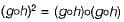Since group is abelian so it is commutative as well as associative.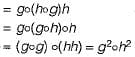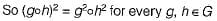Test: Set Theory & Algebra- 2 - Question 2

### Let R be a sym m etric and transitive relation on a set A Then

Detailed Solution for Test: Set Theory & Algebra- 2 - Question 2

A relation which is symmetric and transitive, need not foe reflexive relation.
(i) R = {}; on the set A = {a, b}. The relation R is symmetric and transitive but not reflexive.
(ii) R = {(a, a), (b, b)}; on the set A = {a, b}.
The relation R is symmetric, transitive and also reflexive.
∴ A relation is transitive and symmetric relation but need not be reflexive relation.

Test: Set Theory & Algebra- 2 - Question 3

### Let A and B be sets and let Ac and Bc denote the complements of the sets A and B. The set (A - B) ∪ (B - A) ∪ (A ∩ B) is equal to

Detailed Solution for Test: Set Theory & Algebra- 2 - Question 3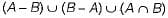Representing above set using Venn diagram as follows: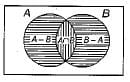∴ By Venn diagram,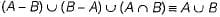Test: Set Theory & Algebra- 2 - Question 4

Which one of the following is false?

Detailed Solution for Test: Set Theory & Algebra- 2 - Question 4

In option (c), the set of all strings over a finite alphabet ∑ doesn’t forms a group under concatenation because the inverse of a string doesn’t exist with respect to concatenation.

Test: Set Theory & Algebra- 2 - Question 5

The binary relation R = {(1, 1), (2, 1), (2, 2), (2, 3), (2, 4), (3, 1), (3, 2), (3, 3), (3, 4)},on the set A = {1 , 2 , 3, 4} is

Detailed Solution for Test: Set Theory & Algebra- 2 - Question 5

The relation R doesn’t contain (4, 4), so R is not reflexive relation.
Since relation R contains ( 1 ,1 ) , (2, 2) and (3, 3).
Therefore, relation R is also not irreflexive.
That R is transitive, can be checked by systematically checking for all (a, b) and (b, c) in R, whether (a, c) also exists in R.

Test: Set Theory & Algebra- 2 - Question 6

Let' P(S) denote the power set of a set S. Which of the following is always true?

Detailed Solution for Test: Set Theory & Algebra- 2 - Question 6

φ always present in any powerset of a set and φ is the only common element between P(S) and P(P(S)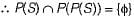Test: Set Theory & Algebra- 2 - Question 7

Consider the set ∑* of all strings over the alphabet ∑ = {0,1}. ∑* with the concatenation operator for strings

Detailed Solution for Test: Set Theory & Algebra- 2 - Question 7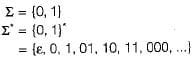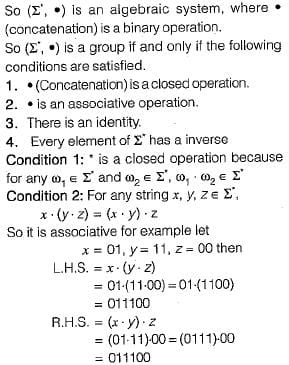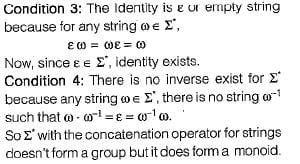Test: Set Theory & Algebra- 2 - Question 8

Consider the binary relation:
S = {(x, y) | y = x + 1 and x, y ∈ {0, 1, 2, ...}}
The reflexive transitive closure of S is

Detailed Solution for Test: Set Theory & Algebra- 2 - Question 8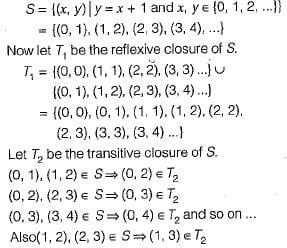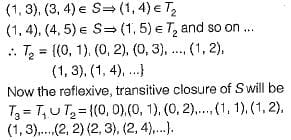Test: Set Theory & Algebra- 2 - Question 9

In a class of 200 students, 125 students have taken Programming Language course, 85 students have taken Data Structures course, 65 students have taken Computer Organization course; 50 students have taken both Programming Language and Data Structures, 35 students have taken both Data Structures and Computer Organization; 30 students have taken both Data Structures and Computer Organization, 15 students have taken all the three courses. How many students have not taken any of the three courses?

Detailed Solution for Test: Set Theory & Algebra- 2 - Question 9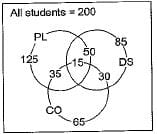According to inclusion - exclusion formula: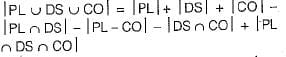PL = students who have taken programming.
DS = Students who have taken Data structures.
CO = Students who have taken Computer Organization.
So, the number of students who have taken atleast 1 of the 3 courses is given by:
= 125 + 85 + 65 - 50 - 35 - 30 + 15
= 175
Therefore, the number of students who have not taken any of the 3 courses is
= Total students-students taken atleast 1 course
= 200 - 175 = 25

Test: Set Theory & Algebra- 2 - Question 10

Let A, B and C be non-empty sets and let X = (A - B ) -C and Y = (A - C) - (B - C)  Which one of the following is TRUE?

Detailed Solution for Test: Set Theory & Algebra- 2 - Question 10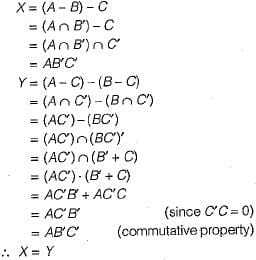Test: Set Theory & Algebra- 2 - Question 11

The set {1, 2, 4, 7, 8,11,13,14} is a group under multiplication modulo 15. The inverses of 4 and 7 are respectively

Detailed Solution for Test: Set Theory & Algebra- 2 - Question 11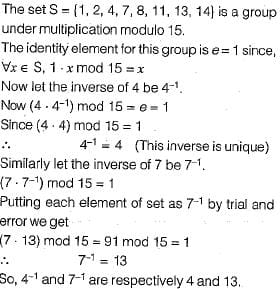Test: Set Theory & Algebra- 2 - Question 12

A relation R fs defined on ordered pairs of integers as follows: (x, y) R (u, v) if x < u and y > v. Then R is

Detailed Solution for Test: Set Theory & Algebra- 2 - Question 12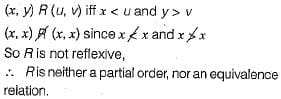Test: Set Theory & Algebra- 2 - Question 13

Let P, Q and R be sets let A denote the symmetric difference operator defined as PΔG = ( P ∪ Q) - (P ∩ Q). Using Venn diagrams, determine which of the following is/are TRUE?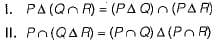Detailed Solution for Test: Set Theory & Algebra- 2 - Question 13

P Δ Q = PQ' + PQ
where Δ is symmetric difference between P and Q.
1.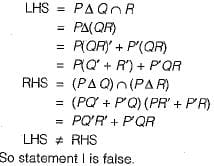2.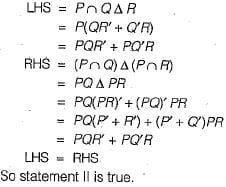Test: Set Theory & Algebra- 2 - Question 14

Consider the binary relation: R = {(x, y), (x, z), (z, x), (z, y)} on the set {x, y, z}. Which one of the following is TRUE?

Detailed Solution for Test: Set Theory & Algebra- 2 - Question 14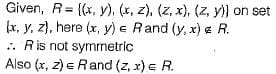∴ R is not antisymmetric.
R is neither-symmetric nor anti-symmetric.

Test: Set Theory & Algebra- 2 - Question 15

How many onto (or surjective) functions are there from an n-element (n ≥ 2) set to a 2-element set?

Detailed Solution for Test: Set Theory & Algebra- 2 - Question 15

Let n = 2. There are only 2 onto functions as shown below: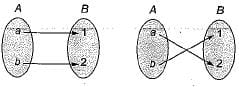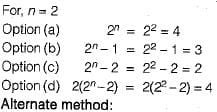The number of onto functions from a set A with m elements to set B with n elements where n < m is given by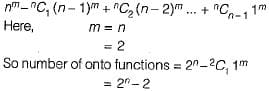## GATE Electrical Engineering (EE) 2024 Mock Test Series

23 docs|285 tests
Information about Test: Set Theory & Algebra- 2 Page
In this test you can find the Exam questions for Test: Set Theory & Algebra- 2 solved & explained in the simplest way possible. Besides giving Questions and answers for Test: Set Theory & Algebra- 2, EduRev gives you an ample number of Online tests for practice

## GATE Electrical Engineering (EE) 2024 Mock Test Series

23 docs|285 tests